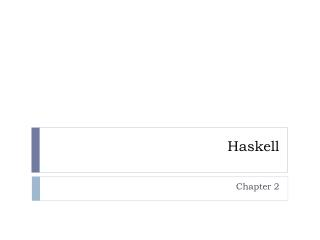Download Presentation# Haskell - PowerPoint PPT Presentation

Haskell. Chapter 2. Chapter 2. Type inference Type variables Type classes. What type is that?. :t expression => type :t ‘a’ ‘a’ :: Char read “a has type of Char” :t [ ] [] :: [a] [] denotes a list tuples have unique types :t (1,2) (1,2) :: (Num t1, Num t) => (t, t1).I am the owner, or an agent authorized to act on behalf of the owner, of the copyrighted work described.
Download PresentationAn Image/Link below is provided (as is) to download presentation

Download Policy: Content on the Website is provided to you AS IS for your information and personal use and may not be sold / licensed / shared on other websites without getting consent from its author.While downloading, if for some reason you are not able to download a presentation, the publisher may have deleted the file from their server.

- - - - - - - - - - - - - - - - - - - - - - - - - - E N D - - - - - - - - - - - - - - - - - - - - - - - - - -
Presentation Transcript
1. Haskell Chapter 2

2. Chapter 2 • Type inference • Type variables • Type classes

3. What type is that? • :t expression => type • :t ‘a’ • ‘a’ :: Char • read “a has type of Char” • :t [ ] • [] :: [a] • [] denotes a list • tuples have unique types • :t (1,2) • (1,2) :: (Num t1, Num t) => (t, t1)

4. Specify type declaration for fn removeNonUpperCase :: [Char] -> [Char] removeNonUppercasest = [c | c <- st, c `elem` ['A'..'Z']] • takes a list of characters, returns a list of characters addThree :: Int -> Int -> Int -> Int addThree x y z = x + y + z • takes 3 integers, returns an integer • Syntax for addThree is covered in chapter 5

5. Common Data Types Type names start with capital letters. • Int. Bounded whole number. • Integer. Really big whole numbers. • Float. Single precision. • Double. Double precision. • Bool. True and False. • Char. Unicode character. • Tuples. Also empty tuple () Don’t begin your function names with upper case!

6. Type variables/Polymorphic types • Some functions can operate on various types (e.g., list functions) :t head head :: [a] -> a • Takes a list of any type, returns that type • Notice the type variable is lower case • Convention is to use 1-character names for type vars :t fst fst :: (a, b) -> a • Notice two different letters, types don’t need to match

7. Type classes • Interface that defines some behavior • If a type is an instance of that type class, then it supports that behavior • If you decide to make a type an instance of a type class, you must define the behaviors associated with that type class. :t (==) (==) :: Eq a => a -> a -> Bool • => is a class constraint • Read as: “The equality function takes two values that are of the same type and returns a Bool. The type of those two values must be an instance of the Eq class.”

8. Common Type Classes • Eq • == and /= • an Eq constraint for a type variable means the function uses == or /= somewhere in its definition* • Ord • Values can be put in some order • >, <, >=,<=,compare • Being an Eq is a prereq for Ord * how does this compare to C++ templates? Java?

9. Common Type Classes, continued • Show • values can be represented as strings • commonly used by show, e.g., show 3 => “3” • cannot “show” a function, e.g., “show doubleMe” is not valid • Read • Takes a string and returns a value whose type is an instance of Read • read "2" + 4.5 • Needs to know what type to return, can’t just do: read "2" • Can use type annotation: read "2" :: Int • Remember: Haskell is statically typed. Doesn’t actually “read” until execution… but type needs to be decided at compile time.

10. Common Type Classes, continued • Enum • Sequentially ordered types • Values can be enumerated • Have defined successors (succ) and predecessors (pred) • (), Bool, Char, Ordering, Int, Integer, Float, Double are Enum • Bounded • minBound and maxBound • maxBound :: Int • type is (Bounded a) => a - polymorphic constants

11. Common Type Classes, continued • Num • numeric, instances act like numbers • :t 20 • 20 :: Num a => a • Also polymorphic constants • :t (*) • (*) :: Num a => a -> a -> a • NOTE: Num a is no longer Show a. Sometimes you need both in the type declaration, e.g., (Num a, Show a) => (a, a) -> a

12. Common Type Classes, continued • Integral • Includes only whole numbers (Int and Integer) • fromIntegral :: (Integral a, Num b) => a -> b • Multiple class constraints (a and b) • Takes an integral value and converts it into more general number • :t length • length :: [a] -> Int • length [1,2,3] + 5.5 – Error! • fromIntegral (length [1,2,3]) + 5.5 • Very useful, because Haskell doesn’t convert integral to floating point automatically. • 8.5

13. Play (no share) • Spend 5-10 minutes • tryout the :t command • play with show and read • play with fromIntegral